# Simple Swept SurfacesGiven two curves C1(u) and C2(v), a swept surface is the surface generated by moving curve C1(v) along curve C2(u). Curve C1(v) may be rotated and scaled. More precisely, for each t in the domain of curve C2(v), curve C1(u) is moved to the point C2(t), possibly with rotation and scaling. Therefore, as t changes from 0 to 1, the transformed curve C1(u) sweeps out a surface and hence the name swept surface. Under this definition, curves C1(u) and C2(v) are referred to as the profile curve and trajectory curve, respectively.

Unfortunately, even though curves C1(u) and C2(v) are both NURBS curves, the generated swept surface may not have a NURBS representation. This will cause problems, since the resulting surface cannot be processed with existing techniques. As a result, this system only supports the generation of swept surface without rotation and scaling. In other word, curve C1(u) only slides along curve C2(v) with the same orientation.

To design a swept surface, select Advanced Features followed by Cross Sectional Design. This will bring up the curve system. In the curve system, one must do the following to obtain a correct swept surface:

1. Design a profile curve.
2. Design a trajectory curve. Note that the profile and trajectory curves do not have to be in the xz- and xy- planes.
3. After adjusting the positions of these two curves, in the curve system, use Curve, followed by Next Curve Segment to make the profile curve the current curve segment.
4. Select Techniques, followed by Generate Swept Surface, followed by Using Identity Matrix. The swept surface defined by these two curves will be shown on the drawing canvas of the surface system.### Example 1: A Swept Cylinder

The easiest swept surface is to have its trajectory curve a line segment. In this case, the swept surface is simply a ruled surface. More precisely, one can create a profile curve, translate it in the direction of the line segment, and construct a ruled surface from these two identical copies of profile curves (but in different locations). The resulting surface is the same as a swept surface. In the following figure, the profile curve is the one used to design a vase in the discussion of surface revolution, and the trajectory curve is a line segment on the x-axis. Click here to download a copy of this file swept-cy.dat.After sending this design to the surface system, we shall see the following swept surface. It is simply a cylinder generating from sliding the vase curve along the line segment.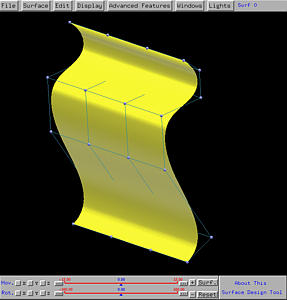### Example 2: A General Swept Surface

If the line segment is replaced with a NURBS curve, the resulting surface will change, because the profile curve will follow the curvature of the trajectory curve. The following example uses the same vase profile, but changes the trajectory curve to a NURBS curve of degree 3. Click here to download a copy of this file swept-surf.dat.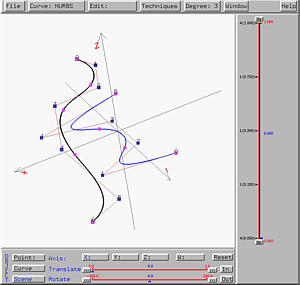As you can see from the following figures, the vase profile follows the curvature given by the trajectory.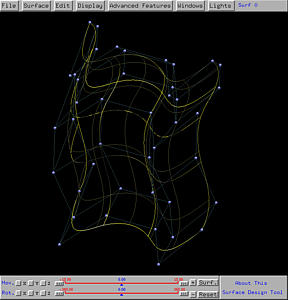### Example 3: A Swept Tube

With swept surfaces, a tube does not have to be linear like a cylinder or circular like a torus. One can use a circle as a profile curve and another curve as a trajectory curve. The following shows a circle in the xz-plane as a trajectory and a NURBS of degree 3 not in the xy-plane as the trajectory. Click here to download a copy of this file swept-tube.dat.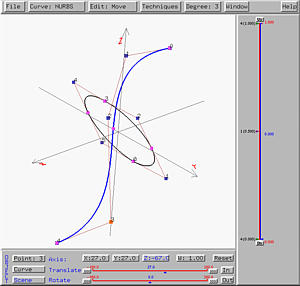From the wireframe figure shown below, you will see that the tube is narrower than the two ends. This is simply because the profile circle moves in parallel with each other and as a result would stack together near the place where the trajectory curve does not change shape "fast enough".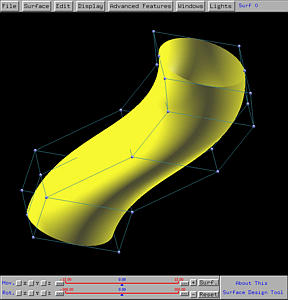### Example 4: A Bad Swept Surface Design

Let us continue with the previous example. We have a profile circle on the xy-plane and a trajectory curve on the xz-plane. Click here to download a copy of this file swept-bad.dat.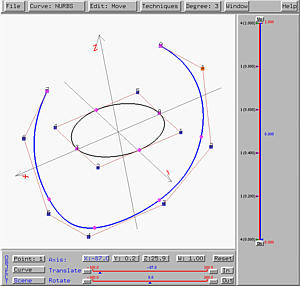The following is the generated swept surface. As you can see from the figure, the bottom part of the surface has a self-intersection. The wireframe figures shows the cause of this self-intersection.